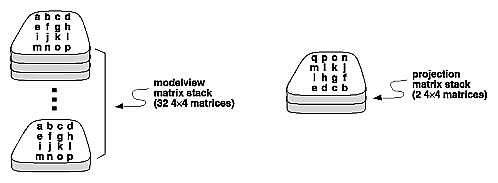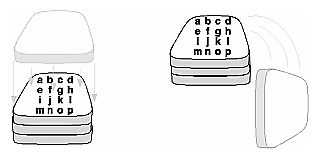## Manipulating the Matrix Stacks

The modelview and projection matrices you've been creating, loading, and multiplying have only been the visible tips of their respective icebergs. Each of these matrices is actually the topmost member of a stack of matrices (see Figure 3-20).Figure 3-20 : Modelview and Projection Matrix Stacks

A stack of matrices is useful for constructing hierarchical models, in which complicated objects are constructed from simpler ones. For example, suppose you're drawing an automobile that has four wheels, each of which is attached to the car with five bolts. You have a single routine to draw a wheel and another to draw a bolt, since all the wheels and all the bolts look the same. These routines draw a wheel or a bolt in some convenient position and orientation, say centered at the origin with its axis coincident with the z axis. When you draw the car, including the wheels and bolts, you want to call the wheel-drawing routine four times with different transformations in effect each time to position the wheels correctly. As you draw each wheel, you want to draw the bolts five times, each time translated appropriately relative to the wheel.

Suppose for a minute that all you have to do is draw the car body and the wheels. The English description of what you want to do might be something like this:

• Draw the car body. Remember where you are, and translate to the right front wheel. Draw the wheel and throw away the last translation so your current position is back at the origin of the car body. Remember where you are, and translate to the left front wheel....

Similarly, for each wheel, you want to draw the wheel, remember where you are, and successively translate to each of the positions that bolts are drawn, throwing away the transformations after each bolt is drawn.

Since the transformations are stored as matrices, a matrix stack provides an ideal mechanism for doing this sort of successive remembering, translating, and throwing away. All the matrix operations that have been described so far (glLoadMatrix(), glMultMatrix(), glLoadIdentity() and the commands that create specific transformation matrices) deal with the current matrix, or the top matrix on the stack. You can control which matrix is on top with the commands that perform stack operations: glPushMatrix(), which copies the current matrix and adds the copy to the top of the stack, and glPopMatrix(), which discards the top matrix on the stack, as shown in Figure 3-21. (Remember that the current matrix is always the matrix on the top.) In effect, glPushMatrix() means "remember where you are" and glPopMatrix() means "go back to where you were."Figure 3-21 : Pushing and Popping the Matrix Stack

void glPushMatrix(void);

Pushes all matrices in the current stack down one level. The current stack is determined by glMatrixMode(). The topmost matrix is copied, so its contents are duplicated in both the top and second-from-the-top matrix. If too many matrices are pushed, an error is generated.

void glPopMatrix(void);

Pops the top matrix off the stack, destroying the contents of the popped matrix. What was the second-from-the-top matrix becomes the top matrix. The current stack is determined by glMatrixMode(). If the stack contains a single matrix, calling glPopMatrix() generates an error.

Example 3-4 draws an automobile, assuming the existence of routines that draw the car body, a wheel, and a bolt.

Example 3-4 : Pushing and Popping the Matrix

`draw_wheel_and_bolts()`
`{`
`   long i;`
` `
`   draw_wheel();`
`   for(i=0;i<5;i++){`
`      glPushMatrix();`
`         glRotatef(72.0*i,0.0,0.0,1.0);`
`         glTranslatef(3.0,0.0,0.0);`
`         draw_bolt();`
`      glPopMatrix();`
`   }`
`}`
` `
`draw_body_and_wheel_and_bolts()`
`{`
`   draw_car_body();`
`   glPushMatrix();`
`      glTranslatef(40,0,30);    /*move to first wheel position*/`
`      draw_wheel_and_bolts();`
`   glPopMatrix();`
`   glPushMatrix();`
`      glTranslatef(40,0,-30);   /*move to 2nd wheel position*/`
`      draw_wheel_and_bolts();`
`   glPopMatrix();`
`   …                    /*draw last two wheels similarly*/`
`}`

This code assumes the wheel and bolt axes are coincident with the z-axis, that the bolts are evenly spaced every 72 degrees, 3 units (maybe inches) from the center of the wheel, and that the front wheels are 40 units in front of and 30 units to the right and left of the car's origin.

A stack is more efficient than an individual matrix, especially if the stack is implemented in hardware. When you push a matrix, you don't need to copy the current data back to the main process, and the hardware may be able to copy more than one element of the matrix at a time. Sometimes you might want to keep an identity matrix at the bottom of the stack so that you don't need to call glLoadIdentity() repeatedly.

### The Modelview Matrix Stack

As you've seen earlier in "Viewing and Modeling Transformations," the modelview matrix contains the cumulative product of multiplying viewing and modeling transformation matrices. Each viewing or modeling transformation creates a new matrix that multiplies the current modelview matrix; the result, which becomes the new current matrix, represents the composite transformation. The modelview matrix stack contains at least thirty-two 4 × 4 matrices; initially, the topmost matrix is the identity matrix. Some implementations of OpenGL may support more than thirty-two matrices on the stack. To find the maximum allowable number of matrices, you can use the query command glGetIntegerv(GL_MAX_MODELVIEW_STACK_DEPTH, GLint *params).

### The Projection Matrix Stack

The projection matrix contains a matrix for the projection transformation, which describes the viewing volume. Generally, you don't want to compose projection matrices, so you issue glLoadIdentity() before performing a projection transformation. Also for this reason, the projection matrix stack need be only two levels deep; some OpenGL implementations may allow more than two 4 × 4 matrices. To find the stack depth, call glGetIntegerv(GL_MAX_PROJECTION_STACK_DEPTH, GLint *params).

One use for a second matrix in the stack would be an application that needs to display a help window with text in it, in addition to its normal window showing a three-dimensional scene. Since text is most easily positioned with an orthographic projection, you could change temporarily to an orthographic projection, display the help, and then return to your previous projection:

`glMatrixMode(GL_PROJECTION);`
`glPushMatrix();                  /*save the current projection*/`
`    glLoadIdentity();`
`    glOrtho(…);                /*set up for displaying help*/`
`    display_the_help();`
`glPopMatrix();`

Note that you'd probably have to also change the modelview matrix appropriately.